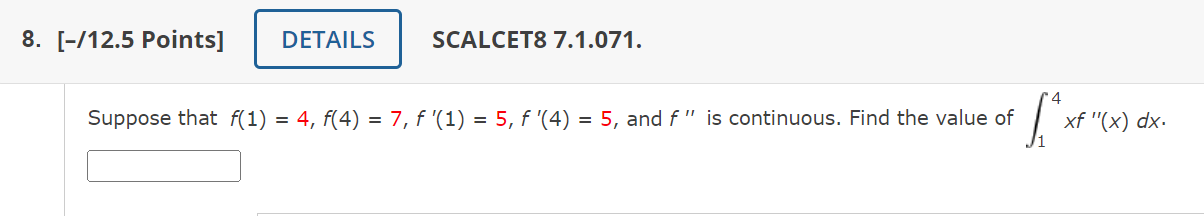Home / Expert Answers / Calculus / suppose-that-f-1-4-f-4-7-f-prime-1-5-f-prime-4-5-and-f-prime-prime-i-pa593

# (Solved): Suppose that $$f(1)=4, f(4)=7, f^{\prime}(1)=5, f^{\prime}(4)=5$$, and $$f^{\prime \prime}$$ i ...Suppose that $$f(1)=4, f(4)=7, f^{\prime}(1)=5, f^{\prime}(4)=5$$, and $$f^{\prime \prime}$$ is continuous. Find the value of $$\int_{1}^{4} x f^{\prime \prime}(x) d x$$.

We have an Answer from Expert